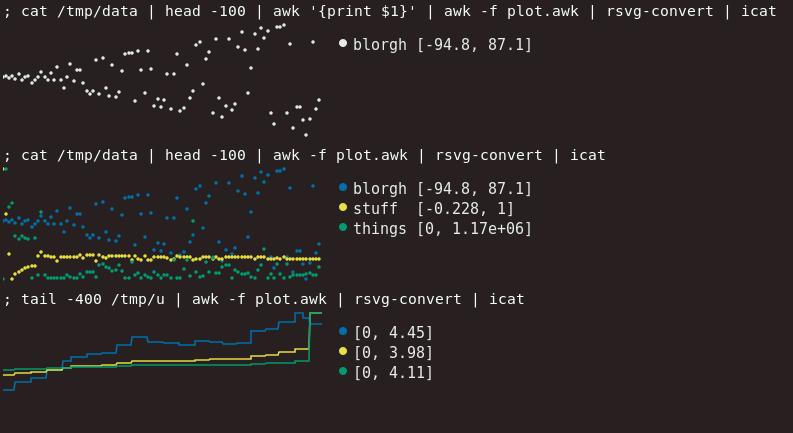Instantly share code, notes, and snippets.

# katef/plot.awk

Last active November 28, 2023 21:42
Star You must be signed in to star a gist
This file contains bidirectional Unicode text that may be interpreted or compiled differently than what appears below. To review, open the file in an editor that reveals hidden Unicode characters. Learn more about bidirectional Unicode characters
 #!/usr/bin/awk -f # This program is a copy of guff, a plot device. https://github.com/silentbicycle/guff # My copy here is written in awk instead of C, has no compelling benefit. # Public domain. @thingskatedid # Run as awk -v x=xyz ... or env variables for stuff? # Assumptions: the data is evenly spaced along the x-axis # TODO: moving average # TODO: trend lines, or guess at complexities # TODO: points vs. lines # TODO: colourblind safe scheme # TODO: center data around the 0 axis # TODO: scanning for all float formats input, including -inf, NaN etc # TODO: guess at whether to use lines or circles, based on delta within a window? function hastitle() { for (i = 1; i <= NF; i++) { if (\$i ~ /[^-0-9.]/) { return 1 } } return 0 } function amax(a, i, max) { max = -1 for (i in a) { if (max == -1 || a[i] > a[max]) { max = i } } return max } function normalise( delta) { for (i = 1; i <= NF; i++) { max[i] = 0 min[i] = 0 for (j = 1; j <= NR; j++) { if (a[i, j] > max[i]) { max[i] = a[i, j] } else if (a[i, j] < min[i]) { min[i] = a[i, j] } } delta[i] = max[i] - min[i] for (j = 1; j <= NR; j++) { a[i, j] -= min[i] if (delta[i] > 0) { a[i, j] /= delta[i] } } } # TODO: rescale to center around 0 # Here the data is squished slightly in descending order of deltas. # Each column is scaled independently anyway, so they're never to scale. # The idea here is to help show intutively which are smaller, but without # actually drawing them to size (since then very small deltas would not be # visible at all). k = 0 prev = -1 while (length(delta) > 0) { i = amax(delta) # Several columns can share the same delta # Formatting to %.3f here is just for sake of rounding if (prev != -1 && sprintf("%.3f", prev) != sprintf("%.3f", delta[i])) { k++ } # +2 to squish things upwards a bit scale = (NF + 2 - k) / (NF + 2) # there's no need to scale by 1 if (scale != 1) { for (j = 1; j <= NR; j++) { a[i, j] *= scale } } prev = delta[i] delete delta[i] } } # internal coordinates to svg coordinates function point(x, y) { x = x * (chart_width - 2 * xmargin) + xmargin y = (height - 2 * ymargin) - y * (height - 2 * ymargin) + ymargin return sprintf("%u,%u", x, y) } function line(i) { printf " \n" } function circles(i) { for (j = 1; j <= NR; j++) { p = point((j - 1) / NR, a[i, j]) split(p, q, ",") printf " \n", q, q, color[i], alpha, color[i], alpha } } function legend_text(i, title) { printf " \n", chart_width + gutter, i * line_height printf " \n", -10, -line_height / 2 + 5, color[i], color[i] printf " %-*s[%.3g, %.3g]\n", sprintf("fill: %s; font-size: %upx; font-family: mono", fg, font_size), (title_width > 0) ? title_width + 1 : 0, title, min[i], max[i] printf " \n" } function display() { print "" printf "" } NR == 1 { if (hastitle()) { for (i = 1; i <= NF; i++) { title[i] = \$i } NR-- next } } { for (i = 1; i <= NF; i++) { a[i, NR] = \$i } } END { fg = "#eeeeee" alpha = "ff" # Bang Wong's colour-safe palette, https://www.nature.com/articles/nmeth.1618 # (using just the last five colours) color = "#009E73" color = "#F0E442" color = "#0072B2" color = "#CC79A7" color = "#D55E00" color = fg if (NF == 1) { color = fg } if (NF > length(color)) { print "too many fields" >> "/dev/stderr" exit 1 } chart_width=320 legend_width=300 height=120 xmargin=0 ymargin=5 gutter=30 font_size=15 line_height=20 # the data is scaled 0..1 for our internal coordinate space normalise() display() }

### katef commented Jul 25, 2020

Optional titles are detected from the data (in TSV format), when present:

``````; head -5 /tmp/data
blorgh stuff things
0 1 0
1.40159 0.495601 1172922
-1.3012 0.0455791 761306
1.69096 -0.227783 800372
``````

Now with (hopefully) colourblind-friendly colours. Colours are only used when there's more than one column to show.### ddunbar commented Nov 22, 2020

Sorry for newb question: `icat` was totally new to me... is this using https://github.com/kovidgoyal/kitty or something else?

### katef commented Nov 25, 2020

Yes, that's a shell alias to `kitty icat`

### Justinzobel commented Apr 13, 2022

Alter lines 151/152 to swap between lines and dots.